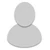## FANDOM

852 Pages

• Which prime numbers satisfy the expression:

666 = p(3·3·3) + p(p(3·3·3))

where p(i) means the ith prime number (that is, p(1) = 2, p(2) = 3, p(3) = 5, etc.)

•